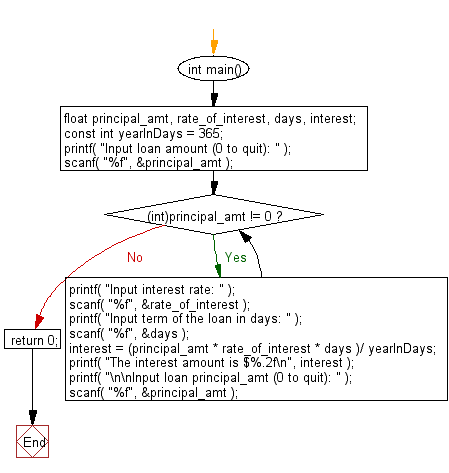﻿ C Program: Calculate the simple interest for the loan - w3resource

# C Exercises: Calculate the simple interest for the loan

## C Basic Declarations and Expressions: Exercise-77 with Solution

Write a C program that accepts principal amount, rate of interest and days for a loan and calculates the simple interest for the loan, using the following formula.
interest = principal * rate * days / 365;

Sample Input: 10000
.1
365
0

Sample Solution:

C Code:

``````#include<stdio.h>

int main()
{
// Declare variables
float principal_amt, rate_of_interest, days, interest;
const int yearInDays = 365; // Constant for converting interest rate

// Prompt user for loan amount
printf( "Input loan amount (0 to quit): " );
scanf( "%f", &principal_amt );

// Main loop for processing loans
while( (int)principal_amt != 0)
{
// Prompt user for interest rate
printf( "Input interest rate: " );
scanf( "%f", &rate_of_interest );

// Prompt user for loan term in days
printf( "Input term of the loan in days: " );
scanf( "%f", &days );

// Calculate interest
interest = (principal_amt * rate_of_interest * days )/ yearInDays;

// Display interest amount
printf( "The interest amount is \$%.2f\n", interest );

// Prompt user for next loan principal_amt
printf( "\n\nInput loan principal_amt (0 to quit): " );
scanf( "%f", &principal_amt );
}

return 0;
}
``````

Sample Output:

```Input loan amount (0 to quit): Input interest rate: Input term of the loan in days: The interest amount is \$1000.00

Input loan principal_amt (0 to quit):
```

Flowchart:C programming Code Editor:

What is the difficulty level of this exercise?

Test your Programming skills with w3resource's quiz.

﻿